﻿ 基于改进GARCH族模型对股市波动率的实证分析 Empirical Analysis of the Volatility of Stock Market Based on the Improved GARCH Model

Vol. 09  No. 02 ( 2020 ), Article ID: 34144 , 11 pages
10.12677/AAM.2020.92017

Empirical Analysis of the Volatility of Stock Market Based on the Improved GARCH Model

Renjie Zhu, Yuanyuan Liu

College of Mathematics and Information Science, Guangxi University, Nanning Guangxi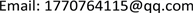Received: Jan. 20th, 2020; accepted: Feb. 3rd, 2020; published: Feb. 10th, 2020ABSTRACT

In view of the fact that the traditional GARCH family model is difficult to describe the stock price volatility of the domestic stock market accurately, in this paper, we firstly improve the original GARCH family model by adding the daily transaction amount in the stock market into the model. Then we use the new model to analyze the stock market of Shanghai and Shenzhen. We also predict the future stock market volatility and finally get better results than the original model.

Keywords:GARCH Model, Stock Market Volatility, Daily Transaction Amount, Volatility Forecast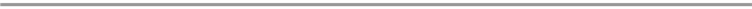1. 引言

2. GARCH族模型的由来及发展

$\begin{array}{l}{r}_{t}={\mu }_{t}+{\epsilon }_{t}\\ {\sigma }_{t}^{2}=\omega +\alpha {\epsilon }_{t-1}^{2}\\ Var〈{\epsilon }_{t}|{I}_{t-1}〉={\sigma }_{t}^{2}\\ {Z}_{t}=\frac{{\epsilon }_{t}}{{\sigma }_{t}}~N\end{array}$

$\begin{array}{l}GARCH模型:\\ {r}_{t}={\mu }_{t}+{\epsilon }_{t}\\ {\sigma }_{t}^{2}=\omega +\alpha {\epsilon }_{t-1}^{2}+\beta {\sigma }_{t-1}^{2}\\ Var〈{\epsilon }_{t}|{I}_{t-1}〉={\sigma }_{t}^{2}\\ {Z}_{t}=\frac{{\epsilon }_{t}}{{\sigma }_{t}}~N\end{array}$ $\begin{array}{l}\text{T-GARCH}模型\\ {r}_{t}={\mu }_{t}+{\epsilon }_{t}\\ {\sigma }_{t}^{2}=\omega +\left(\alpha +\gamma {N}_{t-1}\right){\epsilon }_{t-1}^{2}+\beta {\sigma }_{t-1}^{2}\\ Var〈{\epsilon }_{t}|{I}_{t-1}〉={\sigma }_{t}^{2}\\ {Z}_{t}=\frac{{\epsilon }_{t}}{{\sigma }_{t}}~N\end{array}$

3. GARCH模型族的改进

GARCH族模型的本质是利用已知的股价波动信息拟合出方程去预测股市未来的波动情况。而在GARCH族模型中用到的信息只有 ${\epsilon }_{t-1}$ (与真实值的残差扰动)和 ${\sigma }_{t-1}^{2}$ (上一期的预测方差)，而没有考虑到影响股价波动的其他内在因素，比如：成交金额，股价极差，交易量等。事实上，很多学者的研究已经表明交易量，交易金额对股市的波动具有显著的影响。夏宁伟、詹海、马锋(2014)，先后分别运用深证和上海综合股价指数，沪深300指数，深圳成分指数，证实在中国股票市场中，交易量同样对收益波动率有显著的解释作用，引入交易量的GARCH模型能更好地拟合和预测股票指数的波动性 。而李伶在把交易量引入到GARCH族模型中得到的新的GARCH族模型对股市的分析预测有着比GARCH族模型更好的结果 。本文按照这一思路，将成交金额这一影响因素加入GARCH族模型，得到的改进GARCH模型和改进T-GARCH模型如下：

$\begin{array}{l}改进的\text{GARCH}模型:\\ {r}_{t}={\mu }_{t}+{\epsilon }_{t}\\ {\sigma }_{t}^{2}=\omega +\alpha {\epsilon }_{t-1}^{2}+\beta {\sigma }_{t-1}^{2}+\lambda {S}_{t-1}\\ Var〈{\epsilon }_{t}|{I}_{t-1}〉={\sigma }_{t}^{2}\\ {Z}_{t}=\frac{{\epsilon }_{t}}{{\sigma }_{t}}~N\end{array}$ $\begin{array}{l}改进的\text{T-GARCH}模型：\\ {r}_{t}={\mu }_{t}+{\epsilon }_{t}\\ {\sigma }_{t}^{2}=\omega +\left(\alpha +\gamma {N}_{t-1}\right){\epsilon }_{t-1}^{2}+\beta {\sigma }_{t-1}^{2}+\lambda {S}_{t-1}\\ Var〈{\epsilon }_{t}|{I}_{t-1}〉={\sigma }_{t}^{2}\\ {Z}_{t}=\frac{{\epsilon }_{t}}{{\sigma }_{t}}~N\end{array}$

4. 实证分析

4.1. 数据选取与处理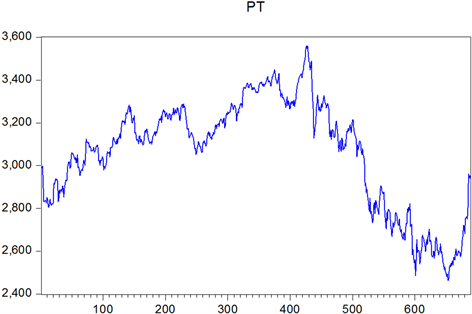Figure 1. Time series chart of Shanghai stock exchange price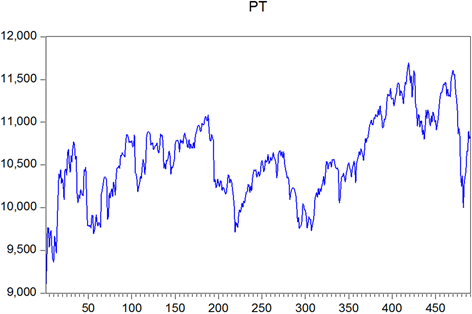Figure 2. Time series chart of Shenzhen stock exchange price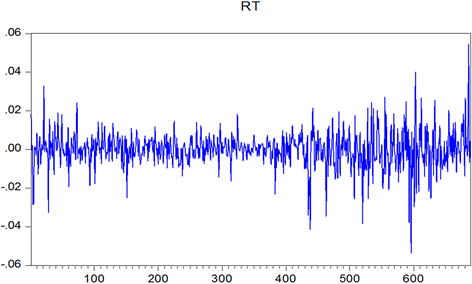Figure 3. Shanghai stock return series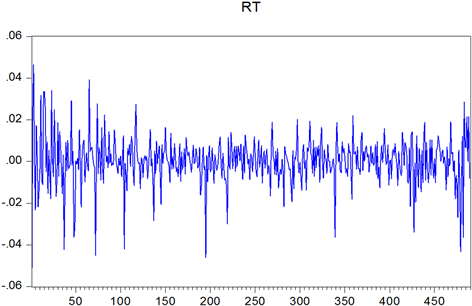Figure 4. Shenzhen stock return series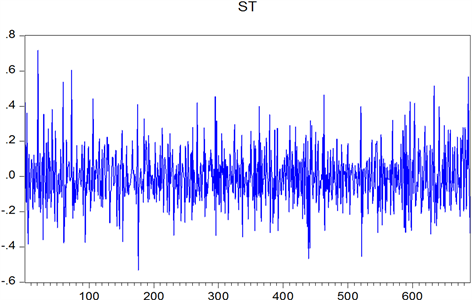Figure 5. Shanghai stock exchange transaction amount series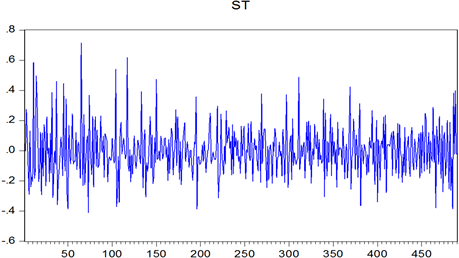Figure 6. Shenzhen stock exchange transaction amount series

4.2. 数据的平稳性以及ARCH效应检验

ARCH是自回归条件异方差的缩写，ARCH效应检验的目的是判断GARCH模型均值方程中残差扰动项平方是否存在自回归的现象，即数据有没有ARCH效应。这是数据能够用GARCH模型族拟合的前提。模型的均值方程为： ${r}_{t}={\mu }_{t}+{\epsilon }_{t}$，记残差的平方为 $\omega$，则有： $\omega ={\left({r}_{t}-{\mu }_{t}\right)}^{2}$，下面对 $\omega$ 作残差平方Q统计量检验，结果见图7图8

4.3. 改进GARCH族模型的拟合Figure 7. Arch test of Shanghai stock exchange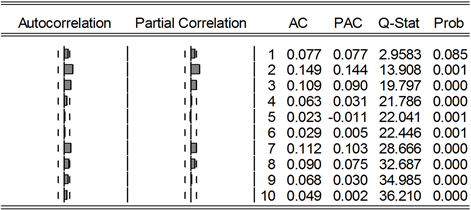Figure 8. Arch test of Shenzhen stock exchange

4.4. 改进GARCH族模型对股市波动的预测

$RMSE=\sqrt{\frac{1}{n}{{\sum }_{t=1}^{n}\left({A}_{t}-{B}_{t}\right)}^{2}}$$MAE=\frac{1}{n}{\sum }_{t=1}^{n}|{A}_{t}-{B}_{t}|$Table 3. Comparison of model prediction ability before and after improvement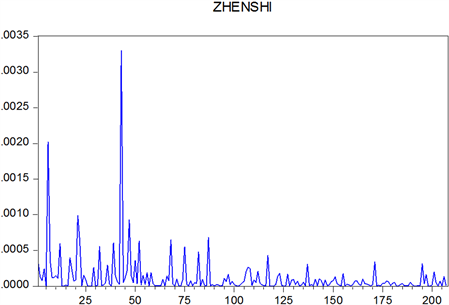Figure 9. Real volatility of Shanghai Stock Index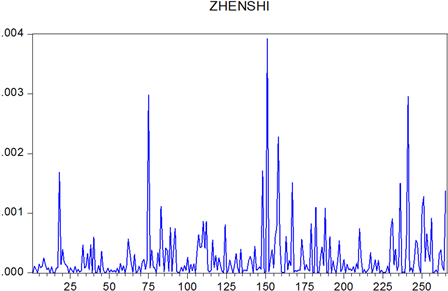Figure 10. Real volatility of Shenzhen Stock Index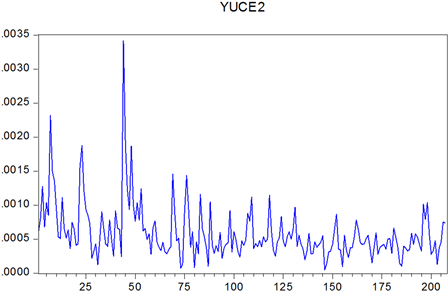Figure 11. Improving GARCH’s forecast volatility of Shanghai StockFigure 12. Improving T-GARCH’s forecast volatility of Shanghai Stock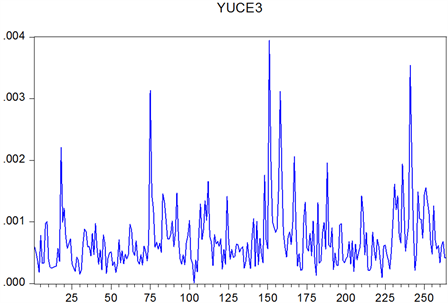Figure 13. Improving GARCH’s forecast volatility of Shenzhen Stock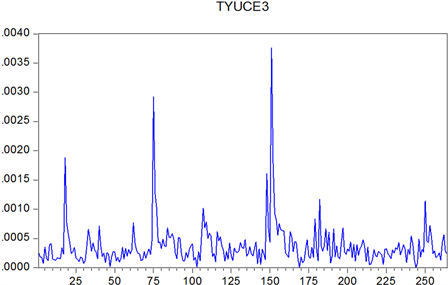Figure 14. Improving T-GARCH’s forecast volatility of Shenzhen Stock

5. 总结

Empirical Analysis of the Volatility of Stock Market Based on the Improved GARCH Model[J]. 应用数学进展, 2020, 09(02): 142-152. https://doi.org/10.12677/AAM.2020.92017

1. 1. Hoch, E., et al. (2019) How Effective and Safe Is Medical Cannabis as a Treatment of Mental Disorders? A Systematic Review. European Archives of Psychiatry and Clinical Neuroscience, 269, 87-105. https://doi.org/10.1007/s00406-019-00984-4

2. 2. 韩莎莎, 张殿杭, 李丽. 大麻二酚的药理研究进展[J]. 药物资讯, 2019, 8(3): 49-55.

3. 3. Ahmed, A.I., van den Elsen, G.A., Colbers, A., et al. (2015) Safety, Pharmacodynamics, and Pharmacokinetics of Multiple Oral Doses of Delta-9-Tetrahydrocannabinol in Older Persons with Dementia. Psy-chopharmacology, 232, 2587-2595. https://doi.org/10.1007/s00213-015-3889-y

4. 4. Van den Elsen, G.A., Ahmed, A.I., Verkes, R.J., Feuth, T., et al. (2015) Tetrahydrocannabinol in Behavioral Disturbances in Dementia: A Crossover Randomized Controlled Trial. The American Journal of Geriatric Psychiatry, 23, 1214-1224. https://doi.org/10.1016/j.jagp.2015.07.011

5. 5. Van den Elsen, G.A.H., Ahmed, A.I.A., Jan Verkes, R., et al. (2015) Efficacy and Safety of Delta-9-Tetrahydrocannabinol in Behavioral Disturbances in Dementia: A Randomized Controlled Trial. Alzheimer’s Dement, 11, 469-470. https://doi.org/10.1016/j.jalz.2015.06.498

6. 6. Allsop, D.J., Copeland, J., Lintzeris, N., Dunlop, A.J., et al. (2014) Nabiximols as an Agonist Replacement Therapy during Cannabis Withdrawal: A Randomized Clinical Trial. JAMA Psychiatry, 71, 281-291. https://doi.org/10.1001/jamapsychiatry.2013.3947

7. 7. Levin, F.R., et al. (2011) Dronabinol for the Treatment of Cannabis Dependence: A Randomized, Double-Blind, Placebo-Controlled Trial. Drug Alcohol Depend, 116, 142-150. https://doi.org/10.1016/j.drugalcdep.2010.12.010

8. 8. Levin, F.R., Mariani, J.J., Pavlicova, M., et al. (2016) Dronabinol and Lofexidine for Cannabis Use Disorder: A Randomized, Double-Blind, Placebo-Controlled Trial. Drug Alcohol Depend, 159, 53-60. https://doi.org/10.1016/j.drugalcdep.2015.11.025

9. 9. Trigo, J.M., Soliman, A., Quilty, L.C., et al. (2018) Nabiximols Combined with Motivational Enhancement/Cognitive Behavioral Therapy for the Treatment of Cannabis Dependence: A Pilot Randomized Clinical Trial. PLoS ONE, 13, e0190768. https://doi.org/10.1371/journal.pone.0190768

10. 10. Trigo, J.M., Lagzdins, D., Rehm, J., et al. (2016) Effects of Fixed or Self Titrated Dosages of Sativex on Cannabis Withdrawal and Cravings. Drug Alcohol Depend, 161, 298-306. https://doi.org/10.1016/j.drugalcdep.2016.02.020

11. 11. Bisaga, A., Sullivan, M.A., Glass, A., et al. (2015) The Effects of Dronabinol during Detoxification and the Initiation of Treatment with Extended Release Naltrexone. Drug Alcohol Depend, 154, 38-45. https://doi.org/10.1016/j.drugalcdep.2015.05.013

12. 12. Jicha, C.J., Lofwall, M.R., Nuzzo, P.A., et al. (2015) Safety of Oral Dronabinol during Opioid Withdrawal in Humans. Drug Alcohol Depend, 157, 179-183. https://doi.org/10.1016/j.drugalcdep.2015.09.031

13. 13. Boggs, D.L., Surti, T., Gupta, A., et al. (2018) The Effects of Cannabidiol (CBD) on Cognition and Symptoms in Outpatients with Chronic Schizophrenia a Randomized Placebo Controlled Trial. Psychopharmacology, 235, 1923-1932. https://doi.org/10.1007/s00213-018-4885-9

14. 14. McGuire, P., Robson, P., Cubala, W.J., et al. (2018) Cannabidiol (CBD) as an Adjunctive Therapy in Schizophrenia: A Multicenter Randomized Controlled Trial. American Journal of Psychiatry, 175, 225-231. https://doi.org/10.1176/appi.ajp.2017.17030325

15. 15. Jetly, R., Heber, A., Fraser, G., et al. (2015) The Efficacy of Nabilone, a Synthetic Cannabinoid, in the Treatment of PTSD Associated Nightmares: A Preliminary Randomized, Dou-ble-Blind, Placebo-Controlled Cross-Over Design Study. Psychoneuroendocrinology, 51, 585-588. https://doi.org/10.1016/j.psyneuen.2014.11.002

16. 16. Cooper, R.E., Williams, E., Seegobin, S., et al. (2017) Canna-binoids in Attention-Deficit/Hyperactivity Disorder: A Randomised-Controlled Trial. European Neuropsychopharmacology, 27, 795-808.https://doi.org/10.1016/j.euroneuro.2017.05.005